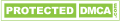# Three Factors that Influence the Accuracy of the Diesel Flue Flow Meter

The detector of diesel flue flow meter has no moving parts and seals. It is not affected by the parameters of the medium, process temperature, process pressure and process density in theory. However, in practice, we find that the accuracy of the measurement is not only affected by the technological conditions of the medium, but also the vibration of the external environment.

(1) The influence of process temperature on the accuracy of the diesel flue flow meter

The quality flow measurement principle of Coriolis diesel flue flow meter is based on the following formula:In the formula: Qm is mass flow;
K is the torsional elastic modulus of the sensing tube;
Δt is the time difference between the left and right sensing tubes;
R is the radius of the left and right sensing tube.

When the K, r is constant, Qm is proportional to the time difference Δt only.

However, Young's modulus of elasticity is a function of temperature. When the temperature changes, the steel of the sensing tube changes accordingly. K is no longer a constant, which affects the accuracy of the diesel flue flow meter; When the temperature changes, it can also cause the imbalance of the geometric structure of the sensor, thus affecting the zero stability of the diesel flue flow meter.

When the flow rate is large, the influence of process temperature change on flow measurement accuracy is not very large. In terms of the CMF200, when rated flow, each change has a degree of accuracy of plus or minus 0.0001%. However, the influence of process temperature on accuracy can not be ignored when the flow rate is small.

(2) The influence of process pressure on the accuracy of diesel flue flow meter

From formula (5), we know that the radius r of the left and right sensing tube changes,  it will also affect the measurement accuracy of the three-free diesel flue flow meter. In practical application, we know that the sensing tube is an elastic element, and the general pipe wall is thinner. When the pressure increases, the r value also increases with increasing, thus affecting the accuracy. As shown in figure 2.From figure 2, we can see the effect of pressure on measurement accuracy: When the process pressure increases, the flow meter can generate a negative deviation, which shows that the flow display value is smaller than the actual value; When the pressure is reduced, the three-free flow meter can generate a positive deviation, which shows that the flow display value is larger than the actual value. On the other hand, the cavitation occurs when the process pressure is equal to or lower than the saturated vapor pressure of the liquid at this temperature. And the metal surface of the sensor tube is damaged by fatigue, which can affect the accuracy and life of the diesel flue flow meter.

(3) The influence of medium density on the accuracy of diesel flue flow meter

It is generally believed that the Coriolis force diesel flue flow meter is not affected by the process density of the medium. But a large number of accurate experimental data and theoretical analysis indicate that this is not the case. For Coriolis force flow meter, the influence of density exists when calibrating and measuring the media of different densities. When a liquid - calibrating diesel flue flow meter is used to measure gases, the error is considerable. The experience has shown that when density changes from 500 kilograms per cubic meter to 1000 kilograms per cubic meter, the effect on accuracy is 0.06%.

Related Articles